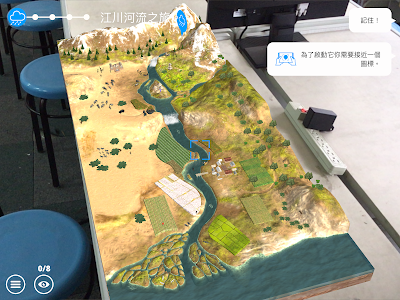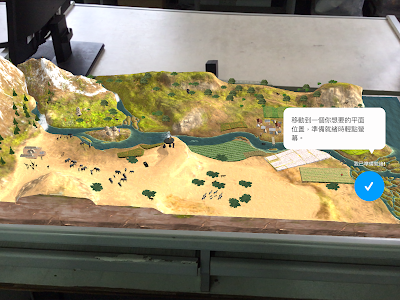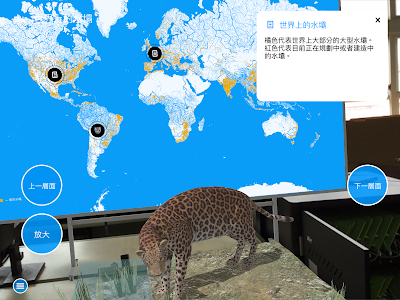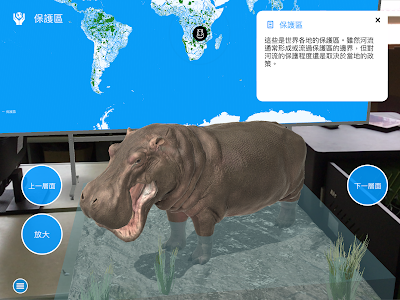## 2018年10月30日

### 用Imagej疊圖看水波干涉的腹線(antinodal line)

1.先用youtube-dl把影片用mp4格式下載回來。
2.用fiji(特製的imagej)的file/import/Movie(FFMPEG)將mp4檔讀成stacks
3.用Image/Stacks/Z Project製作疊圖

Min intensity是把影像中每個隨著時間變化的像素，用最小的數值（相當於最暗的）去疊出來。

## 2018年10月28日

### 使用imagej的Macro切割細胞與細胞追蹤*********************************

setBatchMode(true);
setAutoThreshold("Default");
width = getWidth;
height = getHeight;

n = getSliceNumber();

for (i=n; i<=nSlices-1; i++) {
//for (i=n; i<=n; i++) {
print("==========");
print("i=",i);
roiMan_n = roiManager("count");
print("roiMan_n=",roiMan_n);

run("Select None");
setSlice(i+1);

run("Analyze Particles...", "display add slice");
roiMan_n_new = roiManager("count");
print("roiMan_n_new=",roiMan_n_new);

//計算下一張Slice增加了多少個ROI
roi_add = roiMan_n_new - roiMan_n ;
print("roi_add=",roi_add);

roiManager("Show None");

AND_Big_area= 0; //預設取交集後的面積為零
AND_Big_area_n = 0 ;//預設取交集後的面積，最大的是第零個

for (j=1; j<=roi_add; j++) {
roiManager("Select", newArray(roiMan_n-1,roiMan_n-1+j));
roiManager("AND");
getStatistics(area);
print("j=",j,",area=",area);

//沒交集的面積會回報成整張圖片的面積，所以將回報面積設為0
if(area == width * height){
area =0;
}

if(area>AND_Big_area){
AND_Big_area = area;
AND_Big_area_n = j;
}
}
print("Big=",AND_Big_area_n);
print("AND_Big_area=",AND_Big_area);

//select biggest area and add the new ROI in the next slice
roiManager("Select", roiMan_n -1 + AND_Big_area_n);
roiManager("Add");

//delete all new ROI in the next slice
for (j=1; j<=roi_add; j++) {
roiManager("Select", roiMan_n-1+1);
roiManager("Delete");
}

}

resetThreshold();
setBatchMode(false);

### 使用imagej將影像中的維管束群組化***********************
path = "/home/shawn-pc/桌面/crop/";
//path="c:/users/user/Desktop/crop/";
fileTitle=getTitle;

for (i=0; i<roiManager("count"); ++i) {
roiManager("Select", i);
roiname = call("ij.plugin.frame.RoiManager.getName", i);
run("Duplicate...", "title=crop");
saveAs("Jpeg", path + roiname +".jpeg");
close();
//Next round!
selectWindow(fileTitle);
}

***********************

*************************
//input = "C:/Users/user/Desktop/roi/";
input = "/home/shawn-pc/桌面/imagej竹子維管束/roi/"

particle_size = "70-140";
particle_size_connect= "150-500";
particle_size_horseshoe = "30-200";
particle_size_fragment = "10-100";

particle_cir = "0.6-1.0";
particle_cir_01 = "0.0-1.0";

analy_string = "size="+ particle_size +" circularity="+ particle_cir +" show=Masks clear include summarize add";
analy_string_connect = "size="+ particle_size_connect +" circularity= " + particle_cir_01 +" show=Masks clear include summarize add";
analy_string_horseshoe = "size="+ particle_size_horseshoe +" circularity= " + particle_cir_01 +" show=Masks clear include summarize add";
analy_string_fragment = "size="+ particle_size_fragment +" circularity= " + particle_cir_01 +" show=Masks clear include summarize add";

function single(m){
run("Duplicate...", "title="+m);
run("Analyze Particles...", analy_string);
if(Table.size != 0){
//if ((Table.get("Count",Table.size-1)) != 0) {
roiManager("Save", input + m +".zip");

selectWindow("0");
n=roiManager("count");
for(i=0;i<n;i++){
roiManager("Select", i);
setForegroundColor(255, 255, 255);
run("Fill", "slice");
}
}
n=roiManager("count");
for(i=0;i<n;i++){
roiManager("Select", 0);
roiManager("delete");
}
run("Select None");
roiManager("show none");
close("Mask of "+m);
close("Mask of 0");
close("Mask of Mask of 0");
close(m);

}

function connect(m){
run("Analyze Particles...", analy_string_connect);
run("Watershed");
single(m);
}

//=========================================

name=getTitle;
run("Duplicate...", "title=0");
setAutoThreshold("Default");
run("Convert to Mask");

single(1);

connect(2);

//抓馬蹄形，單獨的
run("Analyze Particles...", "size=70-140 circularity=0.00-1.00 show=Masks clear include summarize add");
run("Close-");
single(3);

//抓馬蹄形，連在一起的
run("Analyze Particles...", analy_string_horseshoe);
run("Close-");
connect(4);

//抓碎片
run("Analyze Particles...", analy_string_horseshoe);
run("Close-");
single(5);

//抓更碎片
run("Analyze Particles...", analy_string_fragment);
run("Close-");
run("Dilate");
run("Fill Holes");
run("Watershed");
run("Erode");
single(6);

//抓更更碎片
run("Analyze Particles...", analy_string_fragment);
run("Dilate");
run("Fill Holes");
run("Erode");
single(7);

list = getFileList(input);
for (i = 0; i < list.length; i++){
roiManager("Open", input+list[i]);

}

selectWindow(name);
roiManager("show none");

roiManager("Show All without labels");

*************************

## 2018年10月12日

### 用在生態系的AR App介紹： WWF Free Rivers

APP一開始先讓你設定模型的位置，你需要一張桌子(或是地板)，然後你就決定模型要擺放的方向。這APP有中文介面，也有中文介紹，畫面就如同下面這樣，是個以河流為主的模型。不過陸域的設定其實是綜合的，看那裏面的動物，同時有熊、獅子、老虎、鱷魚還有白鱀豚...。大概是包含了非洲草原、熱帶雨林、三角洲等等之類的。## 2018年10月8日

### 用QGIS取出海岸線的方法

QGIS作透明的立體等高線地形模型